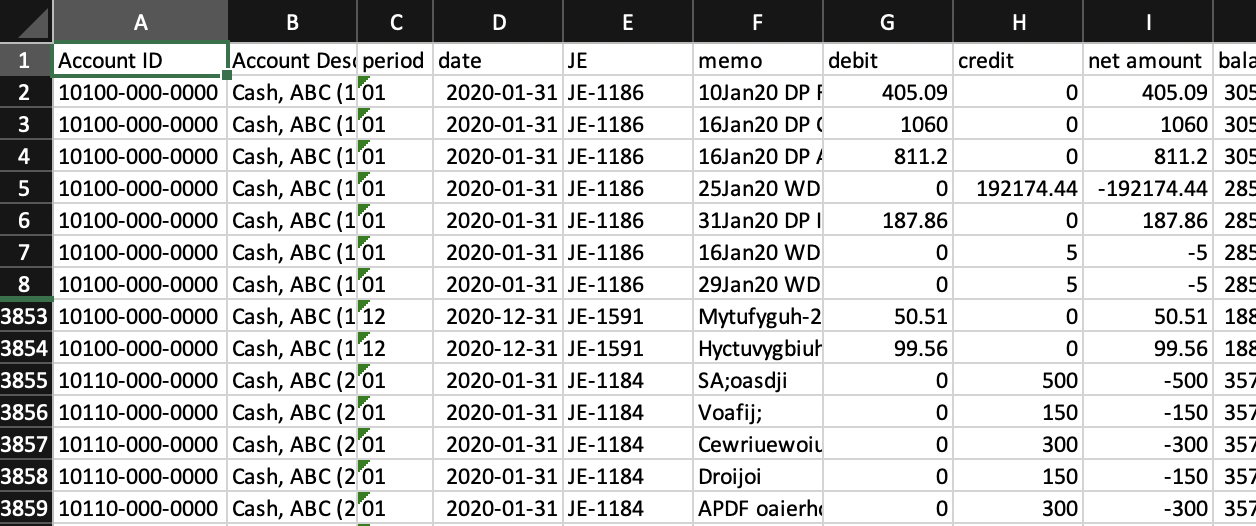# Ungroup and split: Extract complex patternsKate H
• Updated

## Summary

This article will guide you through the process of splitting out more complex sets of information into a usable format.

## Resolution

Consider the following excerpt from a GL file:## The problems:

1. There is no header row (violates overall requirement 1).
2. This file is grouped by account: only one row contains account information (rows 6, 3862), followed by all transactions for that row (rows 8-3860, 3864 to the next GL Account Number) (violates row requirement 1).
3. Account information is in column A but contains "GL Account Number: " followed by account number followed by two space characters followed by account description (rows 6, 3862) (violates column requirement 1).
4. There are unneeded total/subtotal rows (row 3861) and blank rows under the GL Account Number heading that starts an new account (violates overall requirement 2).

## The solution (formatting needed):

1. Add a header row (row 5 is a suitable place to put the column names).
2. Ungroup by account (drag down account information to each transaction row).
3. Split account number and account description into different columns.
4. Exclude extraneous total/subtotal/blank rows and extraneous rows (rows 1-4) above the newly created header row (row 5).

Insert two columns, "Account ID" and "Account Description", before the beginning of the file to split out account number and account description and drag them down.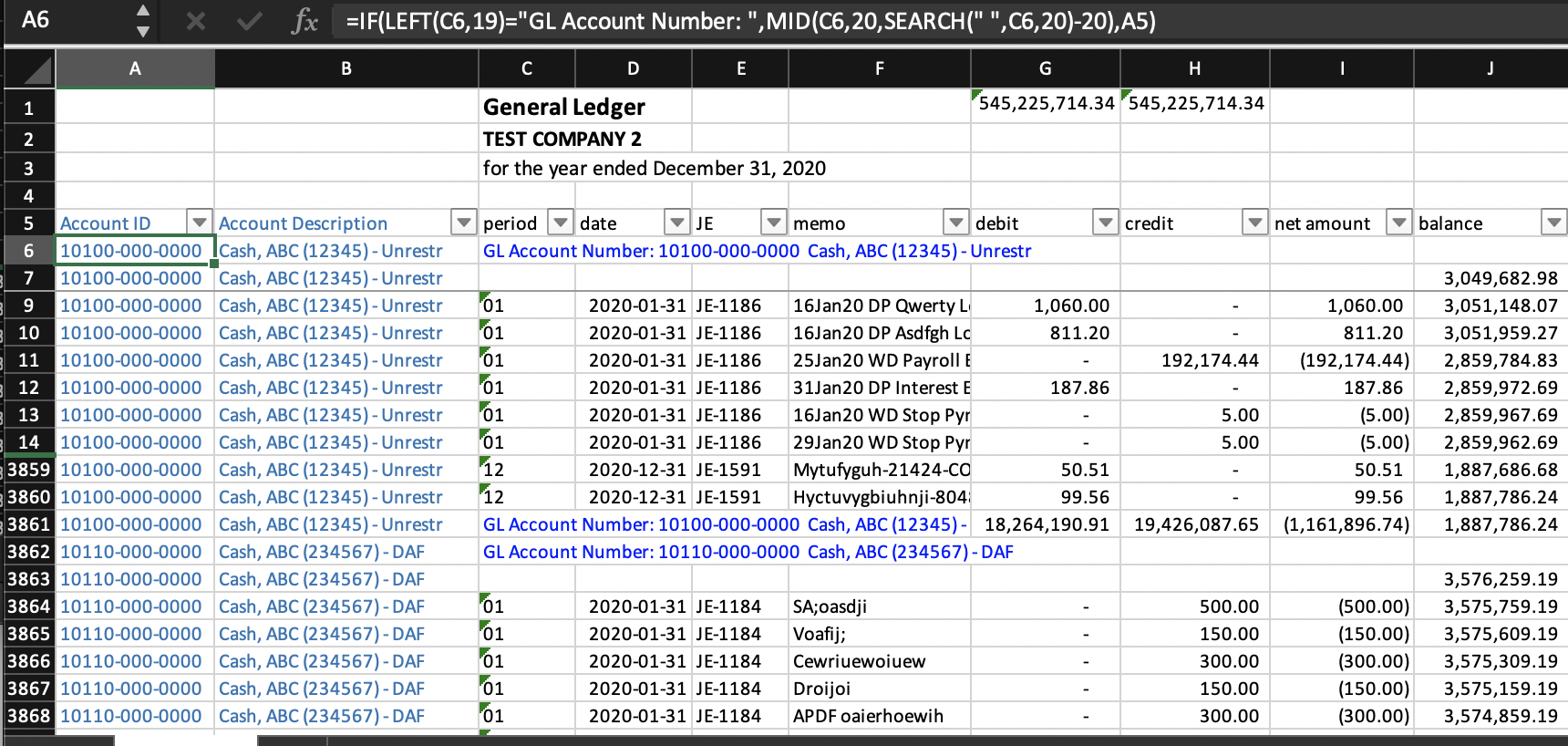### Account ID: Cell A6 and below

`=IF(LEFT(C6,19)="GL Account Number: ",MID(C6,20,SEARCH(" ",C6,20)-20),A5)`
• Account information rows can be identified by column C values that begin with "GL Account Number: ". The 19 in the formula represents the fact that "GL Account Number: " is 19 characters long, and the 20 represents the position where the account number begins (right after the "GL Account Number: ")
• `SEARCH(" ",C6,20)`
finds the position of the first space character in the string C6, starting from position 20 (where the account number begins); in other words, we are trying to find out where the account number ends and the account description begins. Then we subtract 20 from this position to find the length of the account number, to pass to MID() next:
• `MID(C6,20,SEARCH(" ",C6,20)-20)`
is what extracts the account number. The syntax of the MID() function is:
MID(text, start_num, num_chars)
• where text is the string to search (C6)
• start_num is the position to start at (20 because "GL Account Number: " is 19 characters long and the account number begins right after)
• and num_chars is the number of characters to include,
`SEARCH(" ",C6,20)-20`
that is, the length of the account number as discussed above
• IF(LEFT(C6,19)="GL Account Number: ", says to return the account ID (the result of the MID() function above) if C6 begins with "GL Account Number: ", A5 (the calculated cell above) otherwise - this is what does the dragging down (ungrouping) of the account number to all transactions under it.

### Account Description: Cell B6 and below

`=IF(LEFT(C6,19)="GL Account Number: ",TRIM(MID(C6,SEARCH(" ",C6,20)+2,LEN(C6))),B5)`

This column's formulas follow the same logic as that of the Account ID column (column A), except that it extracts the text after the account number (i.e., after the first space after "GL Account Number: "). LEN(C6) ensures that all remaining text to the end is returned.

### Subtotals

The following are the formulas for cells G1 and H1:

`=SUBTOTAL(9,G7:G50000)=SUBTOTAL(9,H7:H50000)`

We use 50000 because this particular file has under 50000 rows. Change that to a large enough number to accommodate the total number of rows your file has. We add these formulas in order to check that the file balances when we do the final step of filtering to keep only the transaction rows (next).

### Filter to keep only transaction rows

After turning on filters, check column D (date) and we see that there are dates in year 2020, and blanks.### Copy and paste values into a new workbook

Noting that all the dates are the transaction rows, select only the dates (uncheck blanks).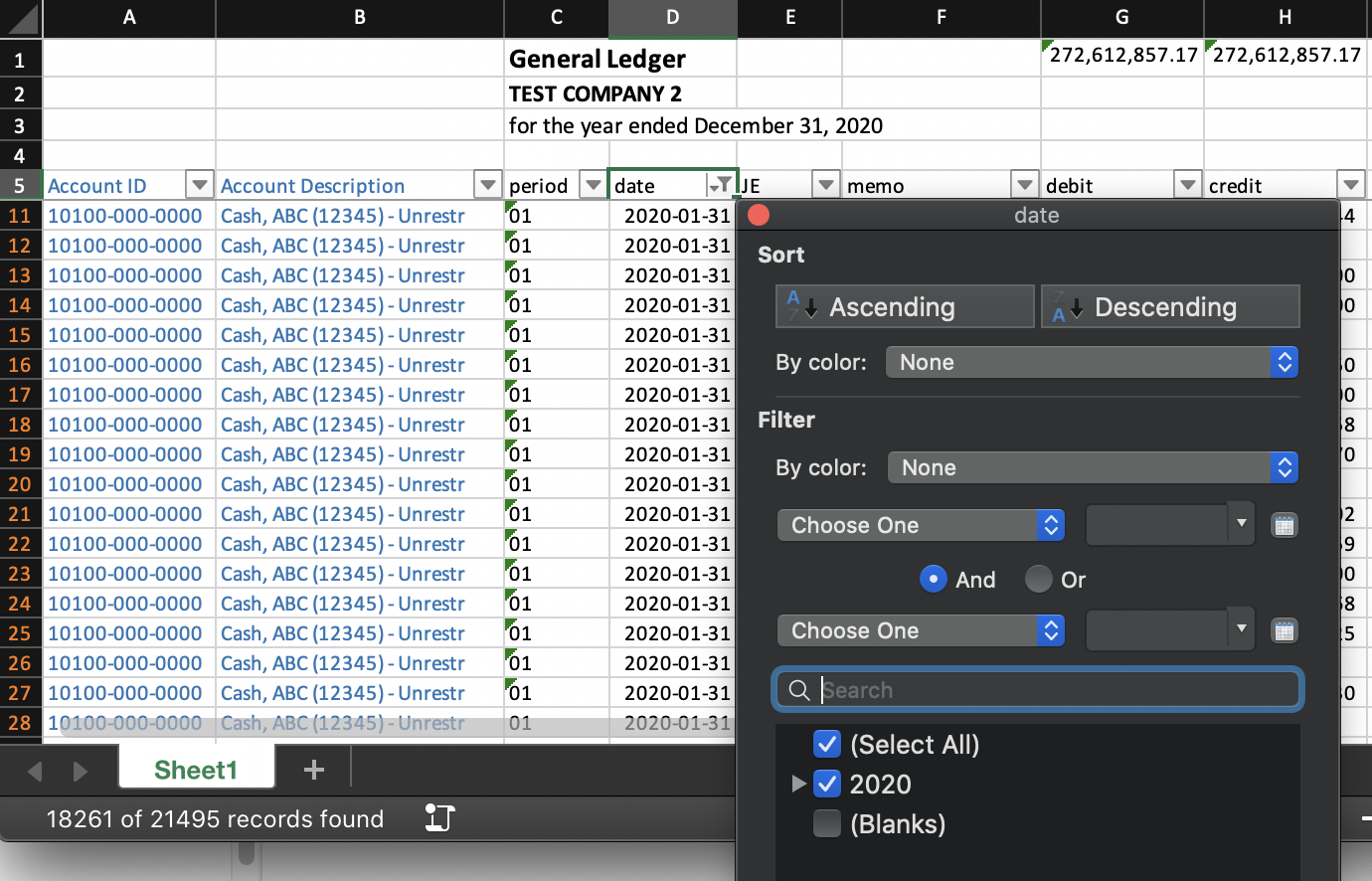Note that results of our SUBTOTAL() formulas in cells G1 and H1 are showing that the total transactions are in balance (yay!)

Our final step is to select the header row and selected transaction rows (i.e., row 5 onward in the filtered view - you can start at cell A5, hit Ctrl-Shift-End to get to the end of the file, and adjust the position as necessary), then copy, then paste special values in a new workbook.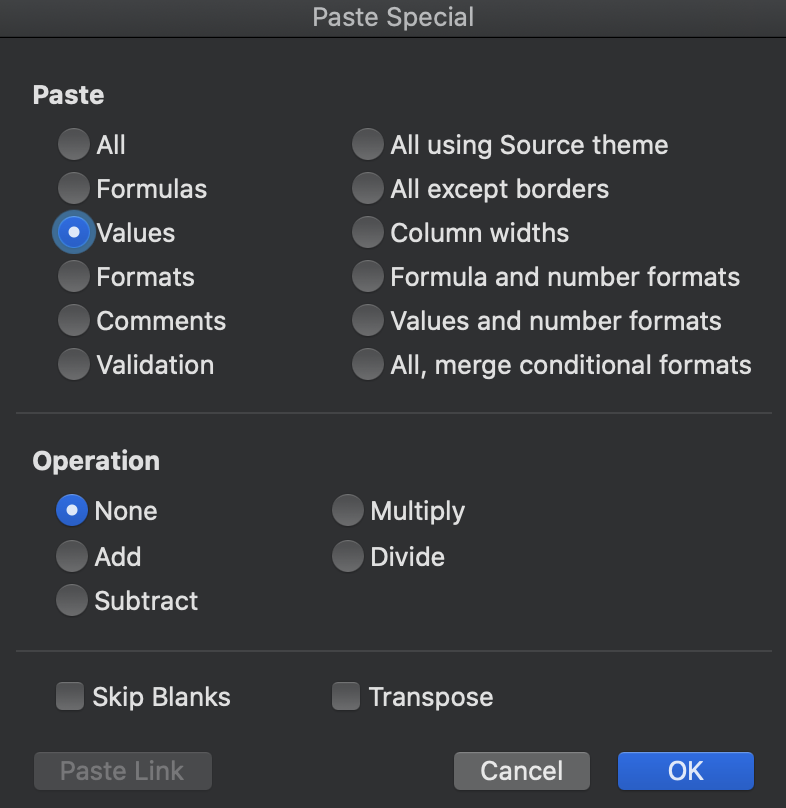Finally, in the new workbook, be sure to format the Date column as Date in YYYY-MM-DD format.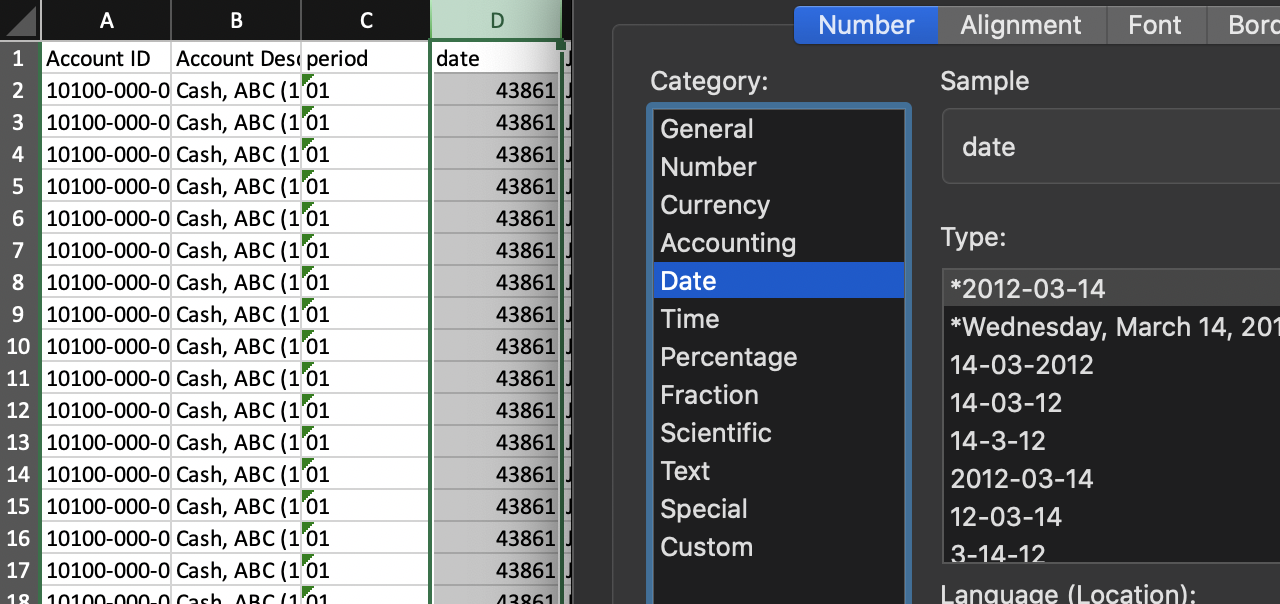And now we have a beautifully formatted file, ready to import into MindBridge!